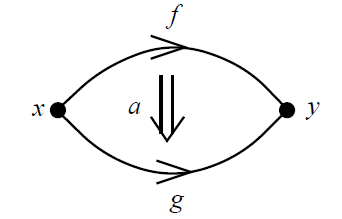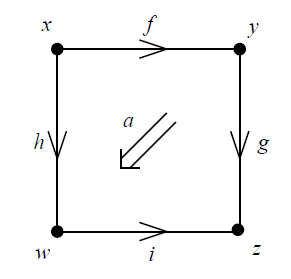Christopher wrote:

> So bicategories are double categories where A and B are the same category?

Not quite, though there's indeed a nice way to get a bicategory from a double category having this property.

Bicategories and double categories provide two ways to enhance the concept of category by including '2-morphisms'. In a bicategory the 2-morphisms are shaped like **bigons**:In a double category the 2-morphisms are shaped like squares:The direction of the double arrow in the middle of this square is unimportant; that's a purely artistic issue.

In a double category the vertical 1-morphisms can be completely different from the horizontal 1-morphisms. In a bicategory there is only one kind of 1-morphism. In both cases there are geometrically plausible ways to compose these 2-morphisms.

There are various ways to get double categories from bicategories and vice versa. Indeed, if you have a double category whose vertical 1-morphisms are exactly the same, with the same rule of composition, as the horizontal 1-morphisms, you can use this fact to get a bicategory with those 1-morphisms.

You can also get a bicategory from _any_ double category, using _another_ obvious trick.

Conversely, Scott mentioned a way to get a double category from a bicategory. Let me enhance his nLab quote slightly:

> If \$$\mathcal{C}\$$ is a bicategory, there is its double category of squares \$$\mathrm{Sq}(\mathcal{C})\$$ whose objects are those of \$$\mathcal{C}\$$, both of whose types of morphisms are the morphisms in \$$\mathcal{C}\$$, and whose squares are 2-morphisms in \$$\mathcal{C}\$$ with their source and target both decomposed as a composite of two morphisms. (These squares are sometimes called *quintets* \$$(\alpha,f,g,h,k)\$$ where \$$\alpha\colon f g \to h k\$$, and so this double category is said to be a quintet construction.)

I recommend this as a very quick and sketchy introduction to such issues; it won't answer all your questions but it will sketch the landscape:

* John Baez, [An introduction to n-categories](http://arxiv.org/abs/q-alg/9705009), in _7th Conference on Category Theory and Computer Science_, eds. Eugenio Moggi and Giuseppe Rosolini, Lecture Notes in Computer Science vol. 1290, Springer, Berlin, 1997.